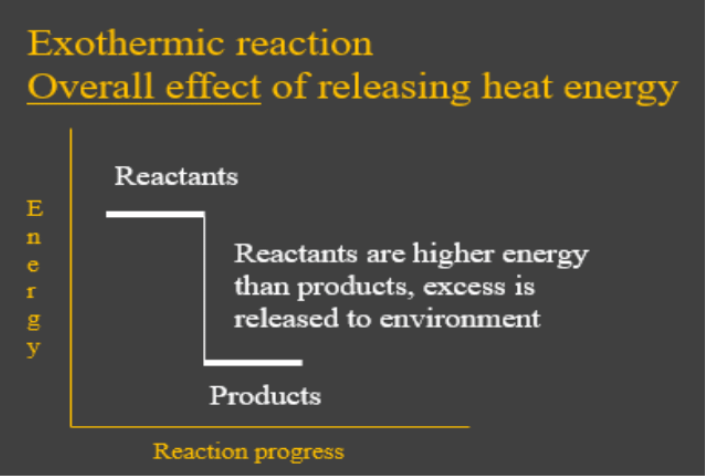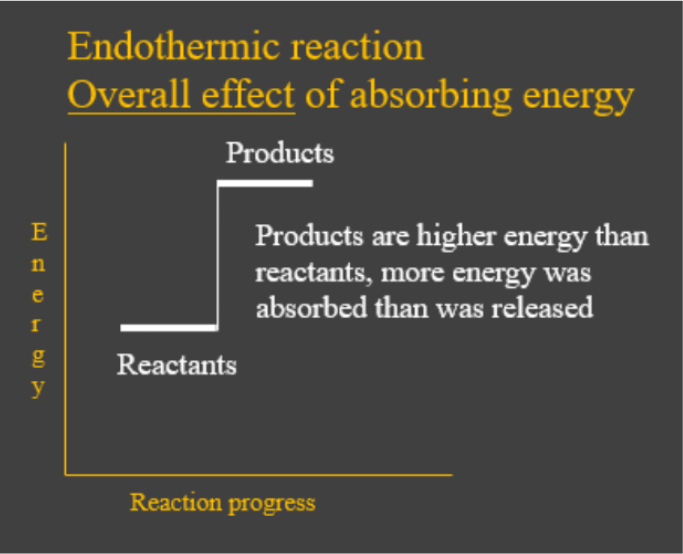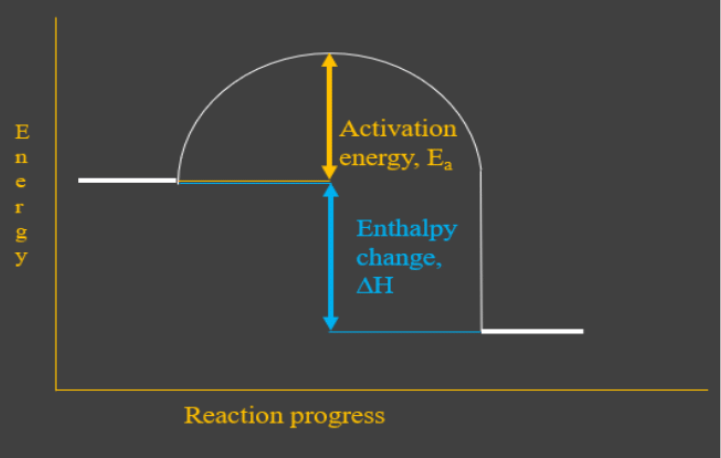# Enthalpy#### Everything You Need in One Place

Homework problems? Exam preparation? Trying to grasp a concept or just brushing up the basics? Our extensive help & practice library have got you covered.#### Learn and Practice With Ease

Our proven video lessons ease you through problems quickly, and you get tonnes of friendly practice on questions that trip students up on tests and finals.#### Instant and Unlimited Help

Our personalized learning platform enables you to instantly find the exact walkthrough to your specific type of question. Activate unlimited help now!##### Intros
###### Lessons
1. Enthalpy changes
2. Exothermic and Endothermic reactions
3. Mean bond enthalpy and other enthalpy definitions.
4. Representing enthalpy changes.
5. Ways to find the enthalpy change.
##### Examples
###### Lessons
1. Recall how to represent the enthalpy change of a reaction.
The reaction between fluorine gas, F2 and hydrogen gas, H2 is as follows. The enthalpy change of the reaction is -539 kJ/mol:

$\mathrm{H_{2(g)} + F_{2(g)} \to 2HF_{(g)}}$

1. i) How can the enthalpy change, ΔH, of the reaction be shown in a reaction equation?
ii) Name one way in which the enthalpy of reaction could be measured.
2. How could this enthalpy change be shown on an energy diagram?
###### Topic Notes

In this lesson, we will learn:

• To understand what is meant by exothermic and endothermic reactions in terms of heat.
• To understand how bond enthalpies are used to find total enthalpy changes in reactions.
• To understand how enthalpy changes are represented in chemistry.
Notes:

• Recall the two ways (mentioned in lessons on Introduction to kinetics) to classify chemical reactions in terms of enthalpy – heat energy of a system:
• Exothermic reactions are chemical reactions which have the overall effect of releasing heat energy to the environment. In other words, the energy put in that was needed to break up the reactant bonds was less than the energy given out when the new bonds in the products formed. This is shown by the energy diagram below:• Endothermic reactions are chemical reactions which have the overall effect of absorbing heat energy from the environment. In other words, the energy given out when the products formed was less than what was needed to break up the bonds in the reactants. This is shown by the energy change diagram below:Note the language overall release/absorbing of energy. All chemical reactions both absorb energy to break reactants and release energy when forming products. The difference between exothermic and endothermic reactions is the amounts in those two steps: exothermic releases more, endothermic absorbs more.

• Energy in those diagrams above means chemical potential energy. This the combined attractive and repulsive forces in the atom or molecule. Attractive forces decrease this potential energy and repulsive forces increase it. This is why high energy molecules are thought of as being less stable than low energy ones.

• It's easiest to think of this in terms of bond breaking and making:
• Ionic and covalent bonds are strong attractive forces that create a stable low energy state. To break those bonds, energy must be put in to bring the bond/molecule to a high energy unstable state and overcome the attractive forces.
Once the bond is broken, the atoms that were making the bond are broken up in an isolated, higher energy state possessing the energy you just put in. We say the process of breaking bonds costs energy.
• When a strong covalent bond (like a C-C bond) forms, think of what the bond is like for the atoms compared to when they are not in a bond. The atoms now have a strong attractive force between them which stabilizes them its a much lower energy state than the high energy, uncombined state before they made the bond. This surplus energy from their old higher energy states is given off to the environment as heat. We say the process of forming bonds releases heat energy to the environment.

• The absolute enthalpy of a substance is hard to measure, and in most cases, we only want to know it so we can find the difference in enthalpy between two substances or groups of substances, like the reactants and products of a reaction. We often just want to know the enthalpy change.
Enthalpy changes are easier to find. If you think of a reaction as breaking some bonds (in the reactants) and forming others (in the products), finding the energy spent doing that is a good place to start!
Mean bond enthalpy is the amount of energy required to break one mole of a chemical bond (one mole of molecules of the bond being talked about). This is usually taken as an average from several similar molecules that contain the bond being investigated.
• This is the easiest measure of a bonds strength. A high bond enthalpy means a lot of energy is required to break this type of bond (for example the NN triple bond or the CO bond).
• This definition is about breaking bonds, so if we are looking at products and we know the mean bond enthalpy of the bonds in the products.

• There are other important enthalpy definitions that should be known:
The standard enthalpy change of reaction, $\Delta H^{\ominus} _{r}$, is the enthalpy change measured when a reaction takes place at standard conditions with all reactants and products in their standard states.
• Standard conditions are defined as 100 kPa and 1M concentration if a solution. 298K is not strictly in the definition but is usually used for more consistency.
• The standard state is the state (solid/liquid/gas) that the substance is most stable and frequently found at under standard conditions, like oxygen gas and liquid water.

The standard enthalpy of reaction is a very general term. Youll normally use a specific version of it for the type of reaction being studied:
• The standard enthalpy of combustion, $\Delta H^{\ominus} _{c}$, is the enthalpy change when one mole of a substance is completely reacted with oxygen at standard conditions.
• The standard enthalpy of neutralization, $\Delta H^{\ominus} _{n}$, is the enthalpy change when an acid and base react to form one mole of water in a neutralization reaction at standard conditions.
• The standard enthalpy of formation, $\Delta H^{\ominus} _{f}$, is the enthalpy change when one mole of substance is formed from its elements in their standard states at standard conditions.

Notice how they all contain one mole and standard conditions? These definitions are consistent so we can easily compare other data. We know if we look in a data book for some values to use in calculation that the values are for doing a reaction with the same amount of substance (one mole) at the same set of conditions (100 kPa, 1M concentration and almost always 298 K).

• Enthalpy change of a reaction can be found by several equations depending on what data you have:

• Using the enthalpy of combustion:

$\Delta H = \Delta H^{\ominus} _{c}$ (reactants) - $\Delta H^{\ominus} _{c}$ (products)

Using the enthalpy of formation:

$\Delta H = \Delta H^{\ominus} _{f}$ (reactants) - $\Delta H^{\ominus} _{f}$ (products)

Using mean bond enthalpy values:

$\Delta H = \sum \Delta H$ (bonds broken) - $\Delta H = \sum \Delta H$ (bonds formed)

Dont get these equations mixed up! The order of products and reactants in each equation is very important and will be looked at more in the next two lessons.

Think about what the sign of the enthalpy change will be from these equations.
The sign of the enthalpy change tells you whether a reaction is exothermic or endothermic:
• A negative $\Delta H$ value means a reaction is exothermic, because the enthalpy of the products is less than the enthalpy of the reactants. This means that the reaction produced lower energy products from higher energy reactants, and the change in heat was released from the system to the surroundings.
• A positive $\Delta H$ value means a reaction is endothermic, because the enthalpy of the products is greater than the enthalpy of the reactants. This means that the reaction produced higher energy products from lower energy reactants, and this net higher energy change was energy absorbed from the surroundings.

• Enthalpy changes for chemical reactions can be presented in a few ways:
• In the form of an equation, for example:

• $A + B +$ 25kJ $\;$$\, C \qquad$ Also written as:
$A + B \;$$\, C \qquad \Delta H = \, +$ 25kJ/mol

• Using an energy change diagram as shown below. The enthalpy change value is marked by the final difference between reactant(s) and product(s) energy.The diagram above is different to those at the top of the page as it includes the activation energy of the reaction.

• There are several ways to measure the enthalpy change of a chemical reaction. Depending on what reaction you are studying, you can use:
• Mean bond enthalpy, (Bond-enthalpy) which is using the sum of bonds broken in reactants against the sum of bonds formed in products to find the enthalpy change.
• Hesss law (Calculating enthalpy: Hesss Law): if you dont know the enthalpy change from $A \,$$\, B$ but you do know it from $A \,$$\, C$ and from $B \,$$\, C$, you can find $A \,$$\, B$ out indirectly.
• Calorimetry (Calorimetry), which measures enthalpy change based on temperature change in a reactions surroundings.